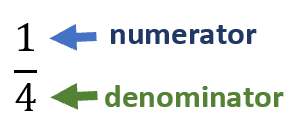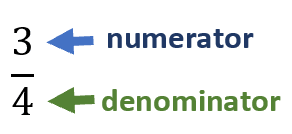# Math Worksheets Land

Math Worksheets For All Ages

# Math Worksheets Land

Math Worksheets For All Ages

# Fractions of Shapes Worksheets

The goal of this section is to help students begin to understand what each portion of a fraction actually represents and make them more real for students. We use well known shapes that students should be very comfortable with them and should be able to partition those shapes into smaller parts. Students will gain several different skills with these worksheets. They will be able to make the clear transition between visuals and numeric values. They will understand the true meaning of the numerator and denominator. If you need a refresher, check the bottom of this page for a full review. These lessons and worksheets help students learn to make observations about fractional shapes.

### Aligned Standard: Grade 3 Geometry - 3.G.2

• Answer Keys - These are for all the unlocked materials above.

### Homework Sheets

I thought up a few new ways to display these models for students.

• Homework 1- Which model displays the fractional values. You will explain them and make numeric values that are equal.
• Homework 2- This is a really review of all the skills that we have explored here.
• Homework 3- There are some really unique shapes here that we have yet to see.

### Practice Worksheets

Students sometimes will struggle with the second problem on each sheet.

• Practice 1- You will need to really understand how to determine the fractions that all these shapes represent.
• Practice 2- Which model displays the fraction 2/6 ? We also ask you about color patterns and arrangement.
• Practice 3- What fraction of the stars are yellow? We also do a problem in hot pink.

### Math Skill Quizzes

Considering the difficult peak on the practice sheets, these seem easy.

• Quiz 1- A good way to see how you are doing with this skill. We focus on understanding bottom heavy fractions.
• Quiz 2- What fraction of the cans are orange? These are most of the exercises that you will see here.
• Quiz 3- Which shape model displays the fraction that is given within each problem.

### What Are Visual Fractions of Shapes?

Every grade elementary teacher has at least once asked the students to visualize their math problems, especially when it comes down to fractions. Fractions are easy to visualize and can be used in everyday life work and activities. They are simply a series of parts that relate to whole. How much of the total whole they are, is indicative of the value of the fraction that they represent. This is a really good way to make fractions tangible. We learn fast when we see things; it helps us remember that action for a longer time. Information becomes easier to comprehend through visual aid. This is the exact purpose of using fractions in a visual form.

Let's remind ourselves the makeup of a fraction. A line exists that splits the two parts of a fraction. The denominator is the bottom number and indicates the number of parts required for one whole unit. The numerator is the top number. This tells us how many parts of the whole that we have.

To understand visual fractions, you need to be able to imagine things while you are reading a math problem. For example, John ate 1/4 of the pizza, how much of the pizza is left?

Is this the image of a pizza that comes to mind?Let's convert that to a mathematical fraction like this:The denominator (4) is the total number of divisions, in this case the total number of slices that makes up an entire pizza. The numerator is how many slices John has. In this case John has 1 of the 4 slices. So, we can say that John has eaten 1/4 of the entire pizza. This does not answer the question that was originally asked, but it has led us to a quick answer. If John ate 1/4 of the pizza that means that 3/4 of the pizza must be left. That is represented by all the remaining slices that you can see. Here is what the fraction that represents the remaining slices would look like.Unlock all the answers, worksheets, homework, tests and more!
Save Tons of Time! Make My Life Easier Now

## Thanks and Don't Forget To Tell Your Friends!

I would appreciate everyone letting me know if you find any errors. I'm getting a little older these days and my eyes are going. Please contact me, to let me know. I'll fix it ASAP.